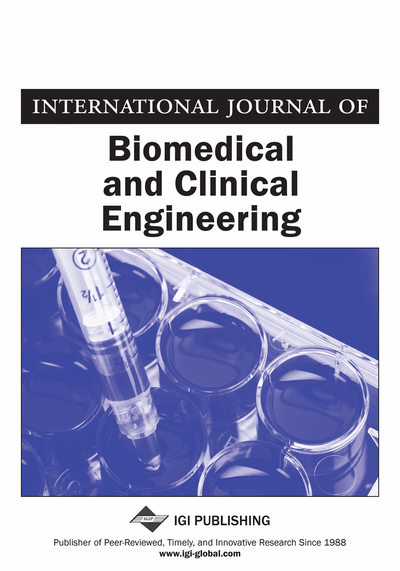# Model Simulating the Heat Transfer of Skin

Anders Jarløv (Department for Medical Engineering, Copenhagen County Hospital, Birkerød, Denmark) and Tim Toftgaard Jensen (Orthopedic Department, Aleris-Hamlet Hospital and Hvidovre Hospital, Birkerød, Denmark)
DOI: 10.4018/IJBCE.2014070104

## Abstract

The relationship between surface temperature and heat transfer in a section of isotropic material with plane parallel sides, in this context simulating human skin, can be attained by means of analogue models. A model, in the form of electric circuits, enables the analogue functions to be recorded as electrical signals. Analogue heat transfer rates derived by processing voltage data from the models can be used for the determination of analogue measures of blood perfusion of the skin, which is generally stated in terms of ml blood flow per 100 ml tissue per minute. Analogue time scales can be compressed, reducing recording times of analogue functions, and inaccessible sites of actual tissues can be made accessible for observation in a model version. Lengthy calculations and the development of formulas can be substituted with the recording of analogue functions. The ability of a perfusable material to simulate the skin model is estimated by how well the material, when subjected to known perfusions with water, exhibit surface temperatures that agree with the corresponding analogue properties of the electrical model. The materials and methods are tentatively applied to recordings of perfusion of human skin.
Article Preview
Top

## Introduction

If one side of a sample of isotropic material with plane parallel sides and an infinite extent is kept at a constant temperature while the other side, which has a lower temperature due to heat dissipation to the environment, is subjected to an instantaneous heat isolation, the temperature on the latter side will rise according to a specific function of time. This temperature course can be simulated in an electric version of an analogue model of the heat transfer in the material, yielding a voltage analogue of the rising surface temperature. The surface temperature will increase faster when the material is additionally perfused with a fluid that has a temperature equal to the constant temperature. Analogue values of perfusions from 0 to 75 ml (100 ml)-1 min-1 are simulated in the electric version of the model where voltages are analogues of temperatures and electric currents are analogues of heat flux. Analogue representations of the thermal properties of materials and of the heat exchange with the environment are represented in the model. The model is expanded to simulate two layers of materials, in this context human skin and underlying fat. Two formulas are used for the retrieval of the analogue values of perfusion, using dynamic voltage recordings as basic data.

The same formulas are used for the retrieval of perfusion rates from surface temperature recordings from a layer of material being subjected to known perfusions with 37 °C water. The perfusions are accomplished using known flow rates to perfuse known volumes of material. The retrieved perfusion rates are compared to the known perfusion rates, and the deviations are presented graphically. Determination of perfusions of human skin in the proximity of the knee joint is demonstrated using procedures similar to those applied with the model experiments.

### Theory

The anatomical properties of human skin and of subcutaneous fat have been described by Wilson, S.B., & Spence, V.A. (1988) and by Loulu, T., & Scott, E. P. (2006). In our study, it is assumed that the thermal properties of skin can be simulated by water saturated porous isotropic layers of materials that have constant thermal parameters. Metabolic heat has been omitted as it is assumed to be constant and of moderate influence on the skin temperature recordings in the present context (Wilson, S.B., & Spence, V.A. (1988)).

Figure 1A shows a sectional view of a layer of isotropic material that simulates the ‘Epidermis’ and ‘Dermis’ that is in direct contact with the constant body temperature. The thickness his assumed to be small compared to the extent of the layer. Blood flow and subcutaneous fat are initially omitted from the figure; only the heat conduction through a single layer is considered.is the temperature in a plane parallel to and at a distancefrom the inner surface. During thermal equilibrium, the temperature, as a function of, is a straight line expressed by the equation:

(1)

When the surface is instantly thermally isolated from the environment, the temperatureis a function of bothand the time.is outlined in Figure 1A and B and must fulfil the one-dimensional fundamental equation for heat transfer (Kittel, C., & Kroemer, H. (2000)):

(2) Where.is the coefficient of heat conduction, ρ is the specific weight of the material and c is the specific heat of the material.is the heat capacity cm-2 surface area., the step response to an instantaneous thermal isolation of the surface, according to Ditlevsen, O (2010), is derived by separating the variablesand, by linear superposition and by the theory of the Fourier series.is expressed by equation (3), which satisfies equation (2), and is specifically established in accordance with the boundary conditions on either side of the material.

(3)

The mathematical foundations are dealt with by Johnsonbaugh, R. & Pfaffenberger, W.E. (2010). and by Klip, W. (1969)

In the following,because only the surface temperature is considered; only the first 2 members of the sequence in equation (3) are applied. Therefore, the error introduced is less than 0.1% offor t>0.06η, where## Complete Article List

Search this Journal:
Reset
Open Access Articles: Forthcoming
Volume 9: 2 Issues (2020): 1 Released, 1 Forthcoming
Volume 8: 2 Issues (2019)
Volume 7: 2 Issues (2018)
Volume 6: 2 Issues (2017)
Volume 5: 2 Issues (2016)
Volume 4: 2 Issues (2015)
Volume 3: 2 Issues (2014)
Volume 2: 2 Issues (2013)
Volume 1: 2 Issues (2012)
View Complete Journal Contents Listing